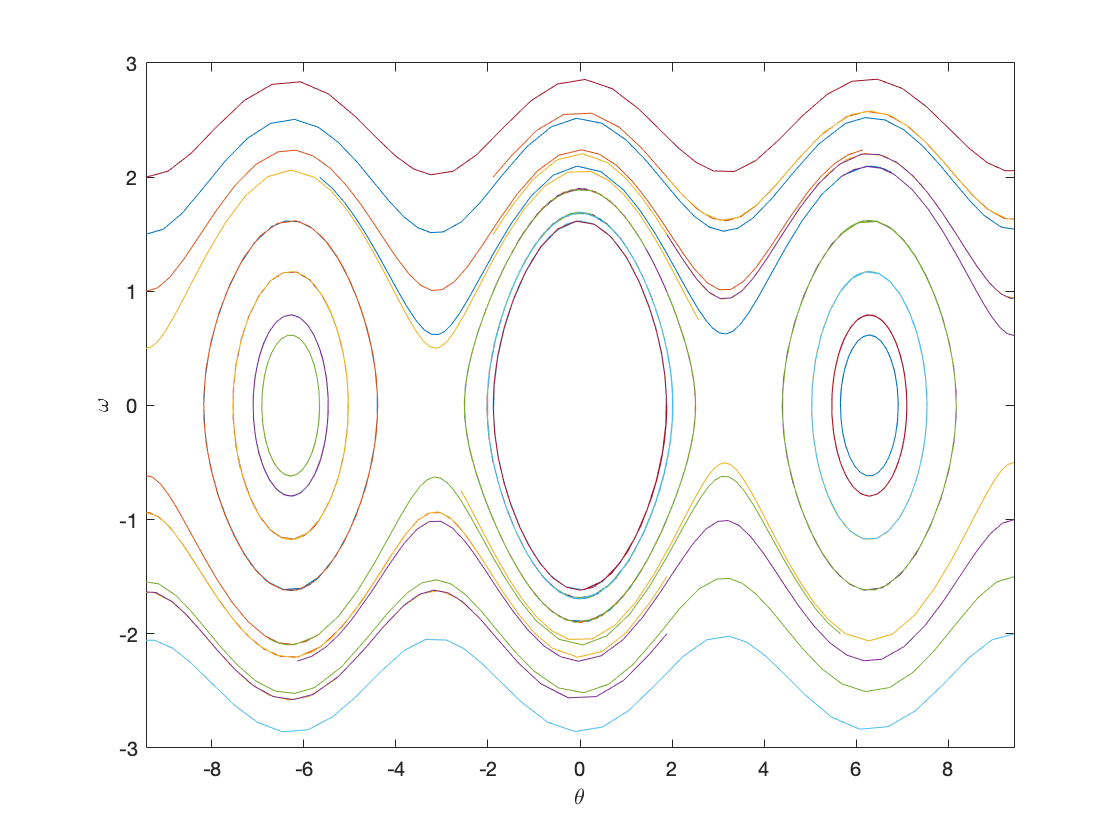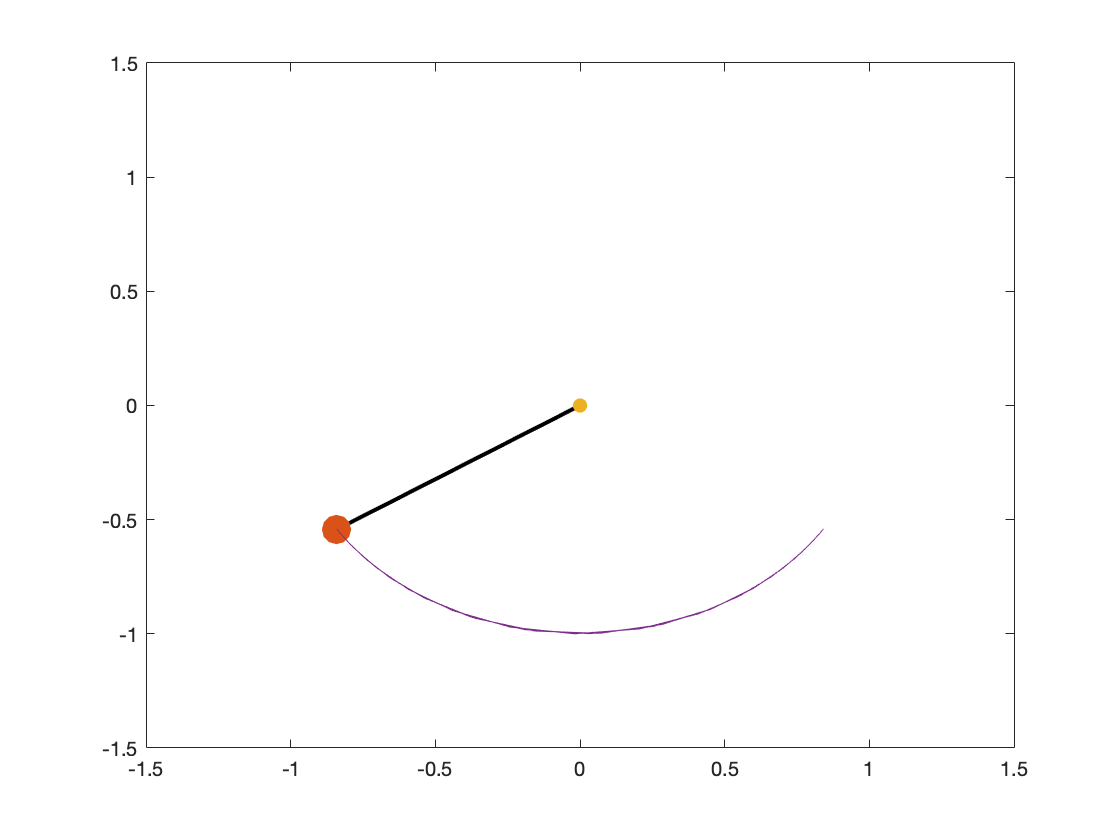# Week 9 Lecture 1: Higher Order ODEs and Dynamical Systems

## Rahman Notes:

Lets's do the examples we did in the theory lecture.
Consider the simple pendulum, which we showed in the theory lecture is modeled asIf we were to use forward Euler, we would get the following scheme:
omega = 0;
theta = 1;
dt = 0.1;
for i = 1:100
theta = theta + dt*omega;
omega = omega + dt*(-sin(theta));
end
format long
theta
theta =
-0.997033921796586
Now lets try it with ode45
omega = 0;
theta = 1;
[T,Y] = ode45(@(t, y) SimplePendulum(t,y),[0 10],[theta omega]);
format long
Y(end,1)
ans =
-0.997381590229234
We can use this to plot our phase plane. We first we need a bunch of initial points, and we let them run.
omega = [-2:0.5:2];
theta = [-3*pi:6*pi/5:3*pi];
for i = 1:length(omega)
for j = 1:length(theta)
[T,Y] = ode45(@(t, y) SimplePendulum(t,y),[0 10],[theta(j) omega(i)]);
plot(Y(:, 1), Y(:, 2))
hold on
end
end
hold off
axis([-3*pi 3*pi -3 3])
xlabel('\theta')
ylabel('\omega')We can even simulate it as a video. Try it for different initial points.
omega = 0;
theta = 1;
[T,Y] = ode45(@(t, y) SimplePendulum(t,y),[0 10],[theta omega]);
y = -cos(Y(:,1));
x = sin(Y(:,1));
for t = 1:length(y)
h = plot([0 x(t)],[0 y(t)],'k',x(t),y(t),'.',0,0,'.',x(1:t),y(1:t));
set(h(1),'linewidth',2);
set(h(2),'MarkerSize',50);
set(h(3),'MarkerSize',20);
axis([-1.5 1.5 -1.5 1.5])
pause(0.1)
endfunction dy = SimplePendulum(t,y) %%% This usually goes at the end of the code.
dy = zeros(2,1);
dy(1) = y(2);
dy(2) = -sin(y(1));
end